Online Tution   »   CBSE Syllabus - Class 9,10, 11,...   »   CBSE Class 11 Maths Syllabus 2022-23...

## Class 11 Maths Syllabus 2022-23

CBSE has announced the CBSE class 11 maths syllabus 2022-23 on its official website. In order to score full marks in Mathematics, the students must plan a strategy to complete CBSE class 11 maths syllabus 2022-23. After completion of CBSE Class 11 Mathematics Syllabus 2023, the students must practice as much as questions they can. The students can assess their preparation by solving previous year’s question papers and practice papers for Class 11 Maths. In this article, we have covered the detailed CBSE class 11 maths syllabus 2022- 2023, Evaluation Scheme, Internal Assessment, Question paper design, and Reference books. The students must go through the whole article and bookmark this page to get the latest updates from CBSE.

## CBSE Class 11Maths Syllabus 2022-23

There are 5 units in CBSE class 11 maths syllabus 2022-23. The units of class 11 maths syllabus 2022-23 are Sets and Functions, Algebra, Coordinate Geometry, Calculus, and Statistics and Probability. Check out the mark distribution given below in the table:

 CBSE Class 11 Maths Syllabus 2022-23 Units No. of Periods Marks Unit 1: Sets and Functions 60 23 Unit 2: Algebra 50 25 Unit 3: Coordinate Geometry 50 12 Unit 4: Calculus 40 08 Unit 5: Statistics and Probability 40 12 Total 240 80 Internal Assessment 20

*No chapter/unit-wise weightage. Care to be taken to cover all the chapters.

## Class 11 Maths Syllabus 2022-23: Unit wise

Here we have given the CBSE Class 11 Mathematics Syllabus 2023 in detail. Every unit is covered in a detailed manner, the students must give importance to the unit which holds higher marks. Check the unit wise CBSE Class 11 Mathematics Syllabus 2023 given below:

## Class 11 Maths Syllabus 2022-23: Unit 1

Unit 1: Sets and Functions

1. Sets: Sets and their representations, Empty set, Finite and Infinite sets, Equal sets, Subsets, Subsets of a set of real numbers especially intervals (with notations). Universal set. Venn diagrams. Union and Intersection of sets. Difference of sets. Complement of a set. Properties of Complement.
2. Relations & Functions: Ordered pairs. Cartesian product of sets. Number of elements in the Cartesian product of two finite sets. Cartesian product of the set of reals with itself (up to R x R x R).Definition of relation, pictorial diagrams, domain, co-domain and range of a relation. Function as a special type of relation. Pictorial representation of a function, domain, co-domain and range of a function. Real valued functions, domain and range of these functions, constant, identity, polynomial, rational, modulus, signum, exponential, logarithmic and greatest integer functions, with their graphs. Sum, difference, product and quotients of functions.
3. Trigonometric Functions: Positive and negative angles. Measuring angles in radians and in degrees and conversion from one measure to another. Definition of trigonometric functions with the help of unit circle. Truth of the identity sin2x + cos2x = 1, for all x. Signs of trigonometric functions. Domain and range of trigonometric functions and their graphs. Expressing sin (x±y) and cos (x±y) in terms of sinx, siny, cosx & cosy and their simple applications. Deducing identities like the following: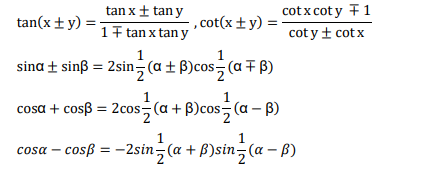Identities related to sin2x, cos2x, tan2 x, sin3x, cos3x and tan3x.

## Class 11 Maths Syllabus 2022-23: Unit 2

Unit 2: Algebra

1. Complex Numbers and Quadratic Equations: Need for complex numbers, especially√−1, to be motivated by inability to solve some of the quadratic equations. Algebraic properties of complex numbers. Argand plane
2. Linear Inequalities: Linear inequalities. Algebraic solutions of linear inequalities in one variable and their representation on the number line.
3. Permutations and Combinations: Fundamental principle of counting. Factorial n. (n!) Permutations and combinations, derivation of Formulae for nPr and nCr and their connections, simple applications.
4. Binomial Theorem: Historical perspective, statement and proof of the binomial theorem for positive integral indices. Pascal’s triangle, simple applications.
5. Sequence and Series: Sequence and Series. Arithmetic Mean (A.M.) Geometric Progression (G.P.), general term of a G.P., sum of n terms of a G.P., infinite G.P. and its sum, geometric mean (G.M.), relation between A.M. and G.M.

## Class 11 Maths Syllabus 2022-23: Unit 3

Unit 3: Coordinate Geometry

1. Straight Lines: Brief recall of two dimensional geometry from earlier classes. Slope of a line and angle between two lines. Various forms of equations of a line: parallel to axis, point  slope form, slope-intercept form, two-point form, intercept form, Distance of a point from a line.
2. Conic Sections: Sections of a cone: circles, ellipse, parabola, hyperbola, a point, a straight line and a pair of intersecting lines as a degenerated case of a conic section. Standard equations and simple properties of parabola, ellipse and hyperbola. Standard equation of a circle.
3. Introduction to Three-dimensional Geometry: Coordinate axes and coordinate planes in three dimensions. Coordinates of a point. Distance between two points.

## Class 11 Maths Syllabus 2022-23: Unit 4

Unit 4: Calculus

1. Limits and Derivatives: Derivative introduced as rate of change both as that of distance function and geometrically. Intuitive idea of limit. Limits of polynomials and rational functions trigonometric, exponential and logarithmic functions. Definition of derivative relate it to scope of tangent of the curve, derivative of sum, difference, product and quotient of functions. Derivatives of polynomial and trigonometric functions.\

## Class 11 Maths Syllabus 2022-23: Unit 5

Unit 5: Statistics and Probability

1. Statistics: Measures of Dispersion: Range, Mean deviation, variance and standard deviation of ungrouped/grouped data.
2. Probability: Events; occurrence of events, ‘not’, ‘and’ and ‘or’ events, exhaustive events, mutually exclusive events, Axiomatic (set theoretic) probability, connections with other theories of earlier classes. Probability of an event, probability of ‘not’, ‘and’ and ‘or’ events.

## Maths Class 11 Syllabus 2022-23: Question Paper Design Based On NCERT

The students studying in Class 11 must know the question paper design. For the understanding of students, the Central Board of Secondary Education has given the question paper design of Mathematics in CBSE Class 11 Mathematics Syllabus 2023. Check out the question paper design given below in the table:

 Typology of Questions Marks Weightage(%) Remembering: Exhibit memory of previously learned material by recalling facts, terms, basic concepts, and answers. Understanding: Demonstrate understanding of facts and ideas by organizing, comparing, translating, interpreting, giving descriptions, and stating main ideas 44 55 Applying: Solve problems to new situations by applying acquired knowledge, facts, techniques and rules in a different way. 20 25 Analysing: Examine and break information into parts by identifying motives or causes. Make inferences and find evidence to support generalizations Evaluating: Present and defend opinions by making judgments about information, validity of ideas, or quality of work based on a set of criteria. Creating: Compile information together in a different way by combining elements in a new pattern or proposing alternative solutions 16 20 Total 80 100
1. No chapter wise weightage. Care to be taken to cover all the chapters
2. Suitable internal variations may be made for generating various templates keeping the overall weightage to different form of questions and typology of questions same.

Choice(s): There will be no overall choice in the question paper. However, 33% internal choices will be given in all the sections

## Maths Class 11 Syllabus 2022-23: Internal Assessment

The internal assessment of Mathematics consists of 20 marks. Internal assessment of mathematics is divided into two parts. Check the details and mark distribution of both the parts given below in the table:

 Internal Assessment Marks Periodic Tests ( Best 2 out of 3 tests conducted) 10 Mathematics Activities 10 Total 20

Conduct of Periodic Tests: Periodic Test is a Pen and Paper assessment which is to be conducted by the respective subject teacher. The format of the periodic test must have questions items with a balanced mix, such as, very short answer (VSA), short answer (SA) and long answer (LA) to effectively assess the knowledge, understanding, application, skills, analysis, evaluation and synthesis. Depending on the nature of subject, the subject teacher will have the liberty of incorporating any other types of questions too. The modalities of the PT are as follows:
a) Mode: The periodic test is to be taken in the form of pen-paper test.
b) Schedule: In the entire Academic Year, three Periodic Tests in each subject may be conducted as follows:

 Test Pre Mid-term (PT-I) Mid-Term (PT-II) Post Mid-Term (PT-III) Tentative Month July-August November December-January

This is only a suggestive schedule and schools may conduct periodic tests as per their convenience. The winter bound schools would develop their own schedule with similar time gaps between two consecutive tests.

c) Average of Marks: Once schools complete the conduct of all the three periodic tests, they will convert the weightage of each of the three tests into ten marks each for identifying best two tests. The best two will be taken into consideration and the average of the two shall be taken as the final marks for PT.

d) The school will ensure simple documentation to keep a record of performance as suggested in detail circular no.Acad-05/2017.

e) Sharing of Feedback/Performance: The students’ achievement in each test must be shared with the students and their parents to give them an overview of the level of learning that has taken place during different periods. Feedback will help parents formulate interventions (conducive ambience, support materials, motivation and morale-boosting) to further enhance learning. A teacher, while sharing the feedback with student or parent, should be empathetic, non- judgmental and motivating. It is recommended that the teacher share best examples/performances of IA with the class to motivate all learners.

## CBSE Maths Class 11 Syllabus 2022-23:NCERT Prescribed Books

Here we have given the reference books for Class 11 Mathematics. These books are given in CBSE Class 11 Mathematics Syllabus 2023, therefore students can solely rely on these books. Check the reference books listed below:

1. Mathematics Textbook for Class XI, NCERT Publications

Related post:

## Maths Syllabus Class 11 CBSE 2022-23: QNA

Q. Will CBSE conduct CBSE Class 11 Exam 2023 annually?
Yes, CBSE has recently announced that from the next session CBSE will conduct annual board exams.
Q. Where I can get CBSE Class 11 Maths Syllabus 2023?
You can get CBSE Class 11 Maths Syllabus 2023 from this page. To get the syllabus of other subjects the students can visit the official website of Adda247 School.
Q. How many units are there in CBSE Class 11 Maths Syllabus 2023?
CBSE Class 11 Maths Syllabus 2023 consists of 5 units. The units of CBSE Class 11 Maths Syllabus 2023 are Sets and Functions, Algebra, Coordinate Geometry, Calculus, and Statistics and Probability.

Sharing is caring!

## FAQs

### Will CBSE conduct CBSE Class 11 Exam 2023 annually?

Yes, CBSE has recently announced that from the next session CBSE will conduct annual board exams

### Where I can get CBSE Class 11 Mathematics Syllabus 2023?

You can get CBSE Class 11 Mathematics Syllabus 2023 from this page. To get the syllabus of other subjects the students can visit the official website of Adda247 School.

### How many units are there in CBSE Class 11 Mathematics Syllabus 2023?

CBSE Class 11 Mathematics Syllabus 2023 consists of 5 units. The units of CBSE Class 11 Mathematics Syllabus 2023 are Sets and Functions, Algebra, Coordinate Geometry, Calculus, and Statistics and Probability.

### TOPICS:

•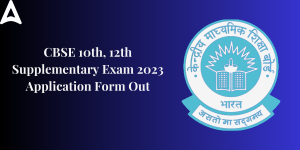CBSE Supplementary Exam 2023, CBSE Compa...
•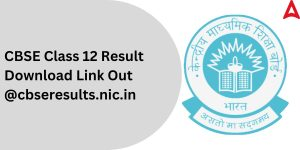CBSE 12th Result 2023 Out, Download link...
•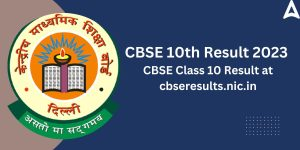CBSE 10th Result 2023 Out, Check CBSE Cl...
•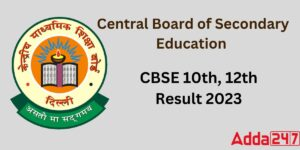CBSE Result 2023 Out, Check Class 12th B...
•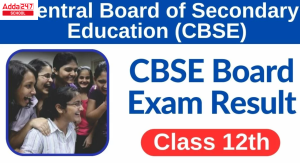CBSE Class 12th Result 2023 Out, Check @...
•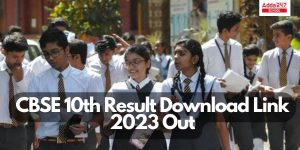CBSE 10th Result 2023 Out, Download Link...